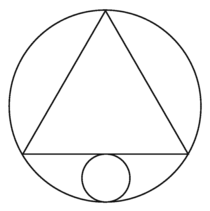# A Triangle Balances on a CircleIn the diagram above, an equilateral triangle is inscribed within a circle, and a smaller circle is inscribed between the two shapes, tangent to both, such that it is the largest possible circle that can be inscribed. If the ratio of the areas of the larger circle to the smaller circle is $a:1$, then find the value of $a$.

×